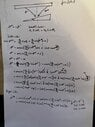# How to compute phase gradient from Snell's law?

• A
• lstnwndrlnd
lstnwndrlnd
TL;DR Summary
I am trying to use Snell's law to derive how to measure phase measurements. I am not sure if the approach I have taken is feasible or if the optical transfer function is a better approach.
I am trying to figure out an intuitive understanding of how differential phase contrast (DPC) as a modality for measuring the phase shift as light passes through transparent samples. In a nutshell, DPC works by using either asymetric illumination or a split detector to standard compound microscope (objective lens, tube lens, camera) and replacing the light source with an LED array. The LED array allows rapid pattern changing for the asymmetric illumination (half circle patterns). Using this technique you can compute the phase gradient of a sample in the x-direction using the following equation: (I_L-I_R)/(I_L+I_R)
where I_L is the intensity image illuminating with the left half of the LED array and I_R is the intensity image illuminating with the right half. It is worth mentioning that in order for this method to work, there must be illumination from outside the numerical aperture of the objective meaning that it requires both brightfield and darkfield illumination to work properly. My understanding of why this is, is because I think that it is obtaining phase information due to refraction of light from the darkfield region, that will enter the objective lens because the light is bent towards the objective. Likewise, brightfield light incident from the other half of the LED array will be refracted away from the objective further increasing contrast.

To study this problem, I am assuming an LED array provides half circle illumination for only two halves (left and right halves) to figure out how this optical configuration plus the equation above can be used to find the gradient in phase in that direction. To simplify the problem, I am assuming a wedge shaped object (so that it has a linear phase gradient in that direction) with an angle, alpha. When alpha is equal to zero, the wedge is just a rectangular prism and the phase gradient is zero. I am also assuming the small angle approximation such that sin(alpha) = alpha, and cos(alpha) = 1.

I have tried a few different approaches to come to a solution, the first of which is using Snell's law. The idea behind using Snell's law is understanding how the refraction of light towards or away from the objective based on this simple sample contributes to obtaining phase information. I attached some math that I have been working on that attempts to predict how the light will change direction between the LED array and the objective lens, but it feels as though this approach is not going anywhere helpful. I know the phase gradient should be phi = deltaN * tan(alpha) = deltaN * alpha because alpha is the slope of the wedge (such that deltaN is the difference in refractive index between the wedge and the surrounding medium). When I have tried to do this, I end up with a bunch of arcsin(lots of stuff) that I can't simplify so it feels like a dead end. Another thing I have been thinking is whether or not phase gradient is something that can be predicted using Snell's Law because my understanding is Snell's Law is a geometric optics way of predicting how light will refract independently of the phase.

The other approach I have seen in an article I read is using the optical transfer function. This seems like a better approach because the optical transfer function does account for both amplitude in phase. In this case the authors suggested the optical transfer function is T = A*exp(i*phi) and when you add the two halves the complex exponential drops out leaving you with A^2 and when you subtract the two halves you end up with A^2 * dphi/dx, and so dividing these two quantities will leave you with the phase gradient dphi/dx. My question about this approach though, is how do I know that T = A*exp(i*phi) is the right right transfer function for my microscope? Also I don't understand how I_L - I_R = A^2 * dphi/dx.

The primary source I have used to get started on this is (I am pretty sure it is open source):
https://opg.optica.org/oe/fulltext.cfm?uri=oe-23-9-11394&id=315599

The other primary source is:
https://iopscience.iop.org/article/10.1088/1361-6463/ac43da

I am not sure if the second one is open source or not so I also attached the page about the theory that outlines the approach using the transfer function. Obviously this is a hard question with no clear solution, so I am mostly asking for any guidance anyone may have in terms of a path forward in trying to better understand the problem.

Thanks!!

#### Attachments

•Image.jpeg
39.9 KB · Views: 18